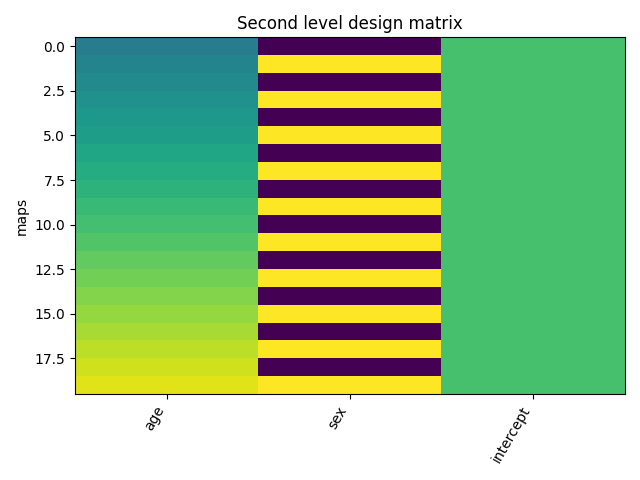# 2.4.1. Example of second level design matrix¶

This example shows how a second-level design matrix is specified: assuming that the data refer to a group of individuals, with one image per subject, the design matrix typically holds the characteristics of each individual.

This is used in a second-level analysis to assess the impact of these characteristics on brain signals.

This example requires matplotlib.

```try:
import matplotlib.pyplot as plt
except ImportError:
raise RuntimeError("This script needs the matplotlib library")
```

## 2.4.1.1. Create a simple experimental paradigm¶

We want to get the group result of a contrast for 20 subjects.

```n_subjects = 20
subjects_label = ['sub-%02d' % i for i in range(1, n_subjects + 1)]
```

Next, we specify extra information about the subjects to create confounders. Without confounders the design matrix would correspond to a one sample test.

```import pandas as pd
extra_info_subjects = pd.DataFrame({'subject_label': subjects_label,
'age': range(15, 15 + n_subjects),
'sex': [0, 1] * int(n_subjects / 2)})
```

## 2.4.1.2. Create a second level design matrix¶

With that information we can create the second level design matrix.

```from nistats.design_matrix import make_second_level_design_matrix
design_matrix = make_second_level_design_matrix(subjects_label, extra_info_subjects)
```

Out:

```/home/kshitij/workspace/nistats-org/nistats-repo/nistats-kchawla-pi/nistats/design_matrix.py:460: UserWarning: Attention: Design matrix is singular. Aberrant estimates are expected.
warn('Attention: Design matrix is singular. Aberrant estimates '
```

Let’s plot it.

```from nistats.reporting import plot_design_matrix
ax = plot_design_matrix(design_matrix)
ax.set_title('Second level design matrix', fontsize=12)
ax.set_ylabel('maps')
plt.tight_layout()
plt.show()
```Out:

```/home/kshitij/workspace/nistats-org/nistats-repo/nistats-kchawla-pi/examples/04_low_level_functions/plot_second_level_design_matrix.py:50: UserWarning: Matplotlib is currently using agg, which is a non-GUI backend, so cannot show the figure.
plt.show()
```

Total running time of the script: ( 0 minutes 0.144 seconds)

Gallery generated by Sphinx-Gallery